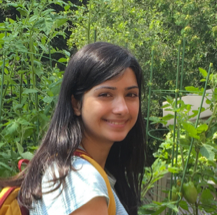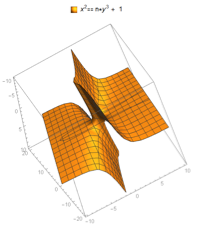#Wolfram Summer School

# Alumni# Nikki Sigurdson

Science and Technology

Class of 2018

## Bio

Nikki Sigurdson is an undergraduate student at the University of Toronto, where she studies computer science and mathematics. She has done research in the field of computer science education, where she has studied the relationship between mathematics and computer science in undergraduate education. She has many interests, including decidability and computability, automata theory and topics in algebra.

## Computational Essay

A Mathematical Exploration through Mathematica with a Problem from Rudin’s Principles of Mathematical Analysis »

## Project: Computational Methods for Analyzing Diophantine Equations

### Goal

This project investigates the solvability of specific cases of Diophantine equations through several forms. Through modern methods in number theory, past case studies of Diophantine equations will be reviewed. Then through an empirical investigation, the scope of decidability in a large set of Diophantine equations will be analyzed for hidden insights.

### Main Results in Detail

Methods for solving Diophantine equations were investigated over a broad range of forms. These forms were chosen based on the selection presented in A New Kind of Science. The results were extended through an empirical study of the smallest solutions to the first 1.02 million Diophantine equations according to the enumeration method designed by M. Szudzik.

### Future Work

This is a difficult subject to study. Empirical investigation has the potential to expose insights about the broad and far-reaching structures underlying the set of these equations. Future work can expand the empirical search in the space of all Diophantine equations and identify threads of decidability that may arise.APPLY NOW